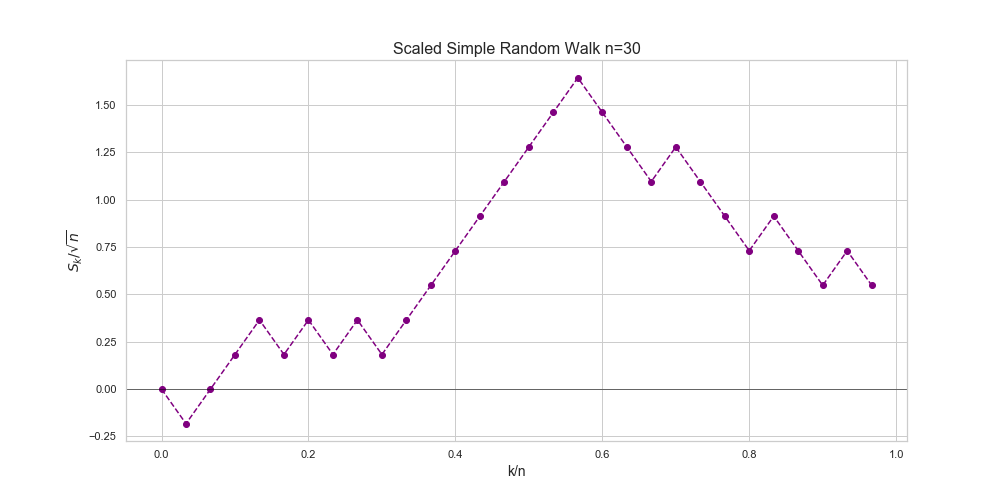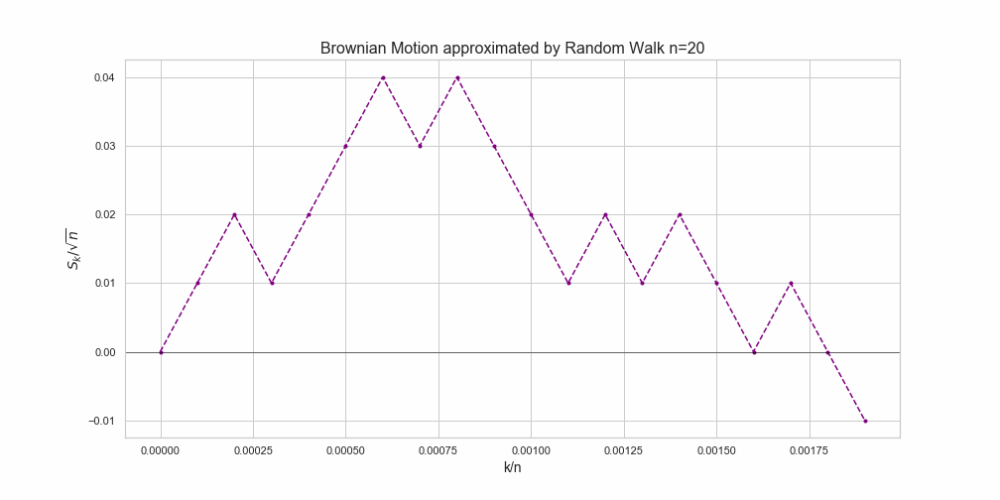## Brownian Motion via Random Walks

The aim of this post is to show how Donsker’s Theorem (also known as Donsker’s Invariance Principle, or the Functional Central Limit Theorem) allows us to simulate paths of the one-dimensional standard Brownian Motion using different kinds of random walks.### 1. Key Concepts

Random Walk – Definition. Let$\{X_i, i \geq 1\}$ be a sequence of real-valued independent an identically distributed (i.i.d.) random variables defined on a probability space$(\Omega, \mathcal{F}, \mathbb{P})$. Then, the stochastic process$\{S_n , n\geq 0\}$, defined as$S_0 =0$, and$S_n = \sum_{i=1}^n X_i, \qquad \forall n\geq 1$,

is called random walk, or more precisely one-dimensional random walked based on$\{X_i, i \geq 1\}$.

Donsker’s Theorem – Let$\{X_i, i \geq 1\}$ be a sequence of real-valued independent an identically distributed (i.i.d.) random variables defined on a probability space$(\Omega, \mathcal{F}, \mathbb{P})$ such that$\mathbb{E}[X_1] =0$, and$\mathbb{V}ar[X_1] =1$. Moreover, let$\{S_n , n\geq 0\}$ be the one-dimensional random walked based on$\{X_i, i \geq 1\}$ and define$\{W^{n}(t), 0\leq t \leq1\}$ as$W^n(0) = 0$, and$W^{n}(t) = \frac{1}{\sqrt{n}} S_{[nt]} + (nt - [nt])\frac{1}{\sqrt{n}}X_{[nt] +1}, \quad t\in(0,1], n\geq 1.$

Then,$W^{n}$ converges weakly to$\mathbb{W}$ as$n \rightarrow \infty$, where$\mathbb{W}$ denotes the one-dimensional standard Brownian motion.

### 2. Some Observations

(1)  In order to understand better this result note that for a given$n$ we have

• For all$t\in [0,1]$ such that$nt$ is an integer,$W^n(t)$ is simply

$W^{n}(t) = \frac{1}{\sqrt{n}} S_{nt}$.

This will be true for all those$t = k/n, k=1, 2, \cdots$ which are in the interval$[0,1]$, i.e.$W^{n}\left( \frac{k}{n} \right) = \frac{1}{\sqrt{n}} S_{k}$.

• For all other$t \in [0,1]$ there is a unique integer$k$ such that$k. Then$W^{n}(t) = W^n\left( \frac{k}{n} \right) + (nt-k)\frac{1}{\sqrt{n}}X_{k+1},$

which can be rewritten as$W^{n}(t) = W^n\left( \frac{k}{n} \right) [1 - (nt-k)] + W^n\left(\frac{k+1}{n} \right)(nt -k).$

This means that  for these points the process$W^{n}$ is simply the linear interpolation between$W^{n}\left( \frac{k}{n} \right)$ and$W^{n}\left( \frac{k +1 }{n} \right)$. As we will see below, this observations makes the simulation (and visualisation) pretty straightforward.

(2) As we mentioned, Donsker’s Theorem is called invariance principle. This is because it tells us that the limiting distribution of the process$W^{n}$ does not depend on the distribution of individual random variables$X_i$ as long as they have zero mean and variance one.

(3) There are several generalisations of this result. The simplest one is to consider random variables with  finite mean and variance, i.e.$\mathbb{E}[X_1] =\mu < \infty$, and$\mathbb{V}ar[X_1] =\sigma <\infty$.

### 3. Visualisation

Now, let’s take a look at the construction of the process$W^{n}$ for a given$n$. Following observation (1) we just need to:

Step 1. Simulate a sample of a sequence of random variables$\{X_k, k=1, \cdots, n \}$ which satisfy the assumptions of Donsker’s Theorem (namely i.i.d with zero mean and variance one) and plot the corresponding random walk, i.e. plot the points$(k, S_k), \qquad k=0,\cdots, n;$

and join them by lines to show the linear interpolation.Step 2. Scale the vertical axis by$\frac{1}{\sqrt{n}}$. That is, plot the points$\left(k, \frac{S_k}{\sqrt{n}}\right), \quad k=0,\cdots, n;$

and join the points by lines to show the linear interpolation.Step 3. Finally, scale the horizontal axis by$\frac{1}{n}$. That is, we plot the points$\left(\frac{k}{n}, \frac{S_k}{\sqrt{n}}\right), \quad k=0,\cdots, n;$

and join them by lines to show the linear interpolation.By Donsker’s Theorem we can say that  for$n$ large enough, our final plot will be a good approximation of a path of the standard Brownian motion on the interval$[0,1]$. Let’s visualise how our approximation looks as$n$ grows from$n=20$ to$n =10000$.The following Python notebook illustrates these steps using random walks based on sequences with different common distributions.